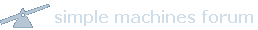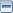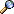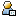Bitcoin ForumAugust 15, 2022, 07:00:56 PMNews: Latest Bitcoin Core release: 23.0 [Torrent]Show Posts Pages: 
 1 Bitcoin / Development & Technical Discussion / Threshold Multisignatures for Bitcoin on: August 28, 2018, 10:25:36 PM Hey, I've been working on a threshold scheme for multisignatures.   I wrote this up, and am pasting it here in the hopes someone can help me find problems with it before I try to bug people on bitcoin-dev again with it and before I try to write up an iacr paper or some nonsense like that (I do think it's nonsense these days...).https://medium.com/@simulx/an-m-of-n-bitcoin-multisig-scheme-e7860ab34e7fThis basically re-uses proven cryptosystems like Schnorr and Shamir secret sharing, and takes advantage of the homomorphic properties of shared secrets in a fairly trivial way that I can't find a problem with.  It also uses the MuSig construction, to prevent participants from being able to birthday-attack parameters of the system.   I don't actually think it's necessary, and I will probably remove it unless someone thinks its needed.An M of N Bitcoin Multisig SchemeCurrently, the leading proposal for a multisignature scheme is an M of M scheme. I would propose a modification of this protocol that provides both aa noninteractive M of M scheme and an interactive but verifiable M of N scheme.Im going to assume that G is a DLP-hard group of some kind, and that curve operations are done using elliptic-curve terminology. EI: Points can be added, and the generator is multiplied by a private key to get a public key.Terminology:G : the agreed upon group (secp256k1)M : the number of participants required to sign.N : the total number of participants. (N≥M)H : a hash function of some kind. (SHA3)P: the order of group GThe public parameters G, M and N must be agreed upon by all participants. If not, consensus verification will fail.M of M SchemeIm going to begin with a description of the M of M scheme. Extending it to M of N requires an application of secret redistribution, which is well reviewed and proven not to leak information about the underlying secrets. I will provide an explanation of how to use it at the end.To initiate a multisig scheme, each of the first M participants generates a secure random number x. Then, each participant i publishes their public key, G*xi.Each party interprets the public keys as shares in a Shamir sharing scheme. Put simply, lagrange coefficients are calculated using Xi = H(G*xi). The coefficients are ordered, based on the sort order of X.`for i1 in sorted(Xis):    coeff[i1]=1    for i2 in Xis:        diff = (i2-i1)%P        inv = modinv(diff, P)        val = i2 *inv%P        coeff[i1]=coeff[i1]*val%P     `These coefficients are used to multiply each point by. The results are summed, and that is the bitcoin public key  the key that these participants are signing for.`for share in shares:    point = coincurve.PublicKey(share.data)    s = point.multiply(coeff[share.index])    vals.append(s)point_sum = coincurve.PublicKey.combine_keys(vals)`So, now we have an M of M public key. Nobody can possibly know the private key, and having M-1 devices gives you zero knowledge of the implied private key x. In addition there is no meaningful way share participants can influence the resulting implied key.We can call this G*x, or the public key in the signing scheme below.Signing:The participants in the scheme can now generate Schorr-style multisignatures.When signing, each participant i rolls a random nonce ki. Each signing participant computes a blinding parameter L. These are akin to those used in the MuSig proposal.`L = H(X1, X2, X3XM, 1)X = SUM(H(L1,Xi))  ### i think this may be unnecessary.   M-1 attackers simply cannot influence either k or x in a meaningful way.R  = G*ke = H(R, M, X)si = ki - xi * e`The signature share is (si, e).Its clear that a single particpant cannot choose a weak public key. In the proposed Shamirs scheme, the value of M-1 shares has zero impact on the entropy contained in the final result. The same logic applies to the implied k. The value of e must be the same for all participants.Verification:The signatures must also be subjected to the lagrange coefficients algorithm above.Each si is combined to a single S by multiplying the coeffs (calculated above) and the result is summed:s = sum(coeff[index] * si)Finally, you perform the same verification as in Schnorr.`rv = G*s + G*x*eev = H(r, M, X1)assert(ev == e)`The math winds up about the same as well. The only variable the attacker controls at signature time is k. M-1 attackers dont affect the randomness of k.Derviation showing this still works.rv = G*(k - x*e) + G(*x*e)rv = G*(k) == ROne thing to understand is that after interpolation, the resulting x and Gx and signatures are combined correctly.Shamir secrets shares are homomorphic in addition, subtraction, multiplication and addition, so this is something we get for free.It is also why ECDSA cant be used in a Shamir scheme. The modular inverse function will not work and shares could not be combined.Addition of Share Participants:Thus far, this scheme can be done without rounds of approvals. Share participants can generate keys offline and without participation from each other.In order to create additional participants, they must be added to a larger implied polynomial using secret redistribution.A quick summary of how this is done doesnt do it justice, but there are lots of implementations and descriptions of secret share redistribution to choose from. For example: https://github.com/dfinity/vssSummary: Each of M participants shards his share into N sub shares labeled 1..N, using Shamirs secret sharing algorithm for an M of N scheme and using random coefficients. These subshares are, further, delivered to each of the corresponding share participants. They are then separately interpolated and combined to form new shares xi . Each participant must then publish the new G*xi. Each participant can now verify that the new combined and implied key G*x for all M subsets of N is the same as the original key G*x. ( Failure to verify this implies that one of the participants is functioning incorrectly and cannot sign  not that there is some sort of weakness. )Redistribution can be used to *remove* participants, *add* them, or otherwise change the M of N scheme without compromising the scheme.Its notable that when using secret redistribution the addition of share participants does not change the verification algorithm, nor does it expose any information about the secret key or the implied secret key.Caveats:Also important to note that this entire article (and multisig schemes in general) assume that there are other mechanisms in place for verifying share participants. Any attacker that controls M of N keys using MITM techniques will be able to sign using any multisig scheme.Public keys should be shared in-person using QR codes or, at least, using verbal or other verification techniques for keys shared online.Currently, the leading proposal for a multisignature scheme is an M of M scheme. I would propose a modification of this protocol that provides both aa noninteractive M of M scheme and an interactive but verifiable M of N scheme.Im going to assume that G is a DLP-hard group of some kind, and that curve operations are done using elliptic-curve terminology. EI: Points can be added, and the generator is multiplied by a private key to get a public key.